# Torsion of a linear connection

This article defines a tensor (viz a section on a tensor bundle over the manifold) of type (1,2)

## Definition

### Given data

• A differential manifold$M$
• A linear connection$\nabla$ on$M$ (viz., a connection on the tangent bundle$TM$).

### Definition part

The torsion of$\nabla$, denoted as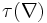$\tau(\nabla)$, is defined as a map that takes as input 2 vector fields and outputs a third vector field, as follows: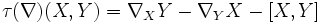$\tau(\nabla)(X,Y) = \nabla_X Y- \nabla_Y X - [X,Y]$

A linear connection whose torsion is zero is termed a torsion-free linear connection.

## Facts

### Tensoriality

Further information: Torsion is tensorial

The torsion map is a$(1,2)$ tensor. It is tensorial in both$X$ and$Y$. This means that the value of the torsion of a connection for two vector fields at a point depends only on the values of the vector fields at that point. In other words,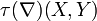$\tau(\nabla)(X,Y)$ at$p$ depends on$\nabla, X(p), Y(p)$ only and does not depend on how$X$ and$Y$ behave elsewhere on the manifold.

### Antisymmetry

Further information: Torsion is antisymmetric

We have that the torsion tensor is antisymmetric, i.e., we have: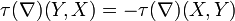$\tau(\nabla)(Y,X) = -\tau(\nabla)(X,Y)$

Equivalently, we have that: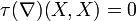$\tau(\nabla)(X,X) = 0$